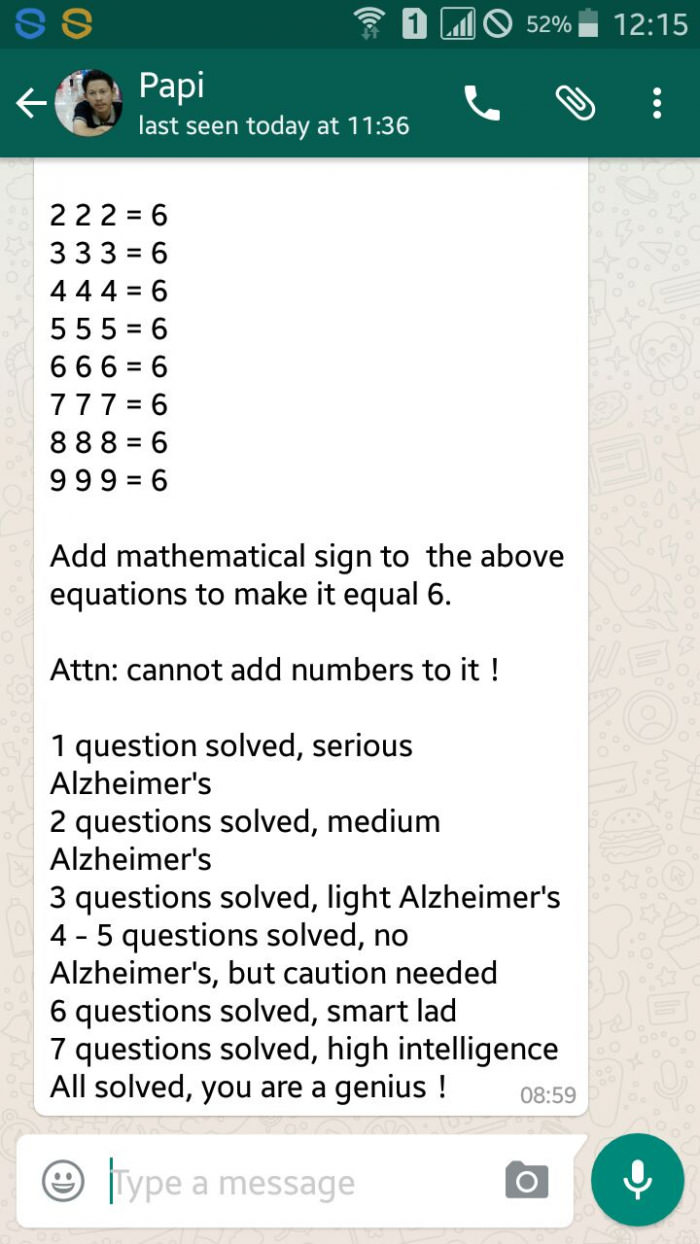# ARE YOU A GENIUS – How Many Equations Can You Solve From This Whatsapp Message??

(Last Updated On: March 1, 2017)## SCROLL DOWN FOR THE POSSIBLE SOLUTIONS

.

.

.

.

.

.

.

.

.

.

.

.

.

.

.

.

.

.

SOLUTIONS TO THE QUESTION ABOVE:

2 + 2 + 2 = 6

3 × 3 -3 = 6

Root 4 + Root 4 + Root 4 = 6

(5/5) + 5 = 6

6 × (6/6) = 6

7 – (7/7) = 6

8 – (root (root (8+8))= 6

(9+9) ÷ (root 9) = 6